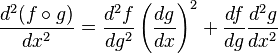# Chain rule for 2nd derivatives

hi does anyone know why the 2nd derivative chain rule is as such?i roughly know that

if u = f(x,y) and x=rcos(T) , y = rsin(T)

then

du/dr = df/dx * dx/dr + df/dy * dy/dr

but if i am going to have a second d/dr, then how does it work out?

## Answers and Replies

I think you're mixing up the chain rule for single- and multivariable functions. For the single variable case,

$$(f \circ g)'(x) = f'(g(x))g'(x)$$

Using the product rule, we then have

$$(f \circ g)''(x) = \frac{d}{dx}[f'(g(x))g'(x)] = f''(g(x)) g'(x) g'(x) + f'(g(x))g''(x) = f''(g(x))[g'(x)]^2 + f'(g(x))g''(x)$$

or in Leibniz notation,

$$\frac{d^2 f}{dx^2}(g(x)) \left[\frac{dg}{dx}\right]^2 + \frac{df}{dx}(g(x)) \frac{d^2 g}{dx^2}$$

For a two-variable function things are more complicated. Suppose we have a function f(x,y) where x and y are themselves functions x(r,t) and y(r,t). As you stated,

$$\frac{\partial f}{\partial r} = \frac{\partial f}{\partial x} \frac{\partial x}{\partial r} + \frac{\partial f}{\partial y} \frac{\partial y}{\partial r}$$

Then

$$\frac{\partial^2 f}{\partial r^2} = \frac{\partial}{\partial r}\left[\frac{\partial f}{\partial x} \frac{\partial x}{\partial r}\right] + \frac{\partial}{\partial r}\left[\frac{\partial f}{\partial y} \frac{\partial y}{\partial r}\right]$$

To make things simpler, let's just look at that first term for the moment. The tricky part is that $\frac{\partial f}{\partial x}$ is still a function of x and y, so we need to use the chain rule again. Using the chain rule,

$$\frac{\partial}{\partial r}\left[\frac{\partial f}{\partial x}\right] = \frac{\partial^2 f}{\partial x^2} \frac{\partial x}{\partial r} + \frac{\partial^2 f}{\partial y \partial x} \frac{\partial y}{\partial r}$$

So

$$\frac{\partial}{\partial r}\left[\frac{\partial f}{\partial x} \frac{\partial x}{\partial r}\right] = \frac{\partial}{\partial r}\left[\frac{\partial f}{\partial x}\right]\frac{\partial x}{\partial r} + \frac{\partial f}{\partial x} \frac{\partial^2 x}{\partial r^2} = \left(\frac{\partial^2 f}{\partial x^2} \frac{\partial x}{\partial r} + \frac{\partial^2 f}{\partial y \partial x} \frac{\partial y}{\partial r}\right)\frac{\partial x}{\partial r} + \frac{\partial f}{\partial x} \frac{\partial^2 x}{\partial r^2}$$

I'm feeling a bit too lazy at the moment to write out the whole thing for $\frac{\partial^2 f}{\partial r^2}$. (So many \frac's and \partial's...). Hopefully what I've written so far will help you enough and hopefully I didn't make any mistakes!

ah i see... thank you very much